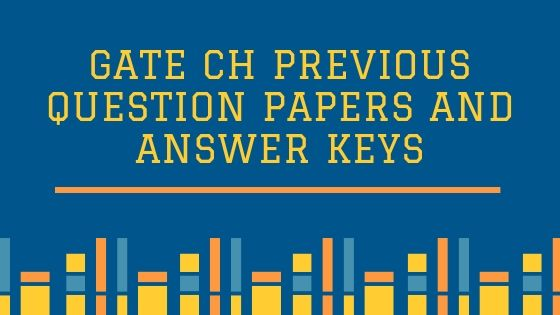GATE

# Last 10 Year question papers and keys of GATE CH

### GATE Chemical Engineering Previous Papers collectionGraduate Aptitude Test in Engineering (GATE) is an examination conducted by the Indian Institute of Science (IISc), Bangalore along with seven Indian Institutes of Technology on behalf of MHRD and Government of India to test the comprehensive understanding of the candidates in various undergraduate subjects related to Science.

GATE Qualification is mandatory for seeking admission to:
(i) Master’s programs in Engineering/ Architecture/ Technology
(ii) Doctoral programs in relevant branches of Science, in the institutions supported by the MHRD and other Government agencies.

The score of GATE Examination is valid for Three Years from the announcement of the results and they are accepted by more than 900+ institutes across India.

A collection of previous years GATE CH Question papers with answers are provided below to give a better understanding about GATE CH upcoming examination.

For latest updates on GATE 2020, visit http://gate.iitm.ac.in/

### The key points about GATE CH exam are:

• The exam is conducted ONLINE
• It is a duration of 3 hours.
• Questions are divided into three sections: General Aptitude, Engineering Mathematics, and Subject-specific part.
• Number of questions – 65 totaling to 100 marks
• Question type: Multiple Choice Questions (MCQs) and Numerical Answer Type (NAT) Questions. Of 1 mark and 2-marks respectively.
• Marking scheme: MCQs carry negative marks, while NAT questions don’t attract negative marking.

Here is a brief on the topics covered for GATE 2020 Chemical Engineering examination.

## GATE CH Syllabus:

Section 1: Engineering Mathematics

Linear Algebra: Matrix algebra, Eigen values and eigenvectors.

Calculus: Taylor series, Mean value theorems, Stokes, Gauss and Green’s theorems. Differential equations: Cauchy’s and Euler’s equations, Initial and boundary value problems, Laplace transforms and Laplace equation.

Complex variables: Complex number, polar form of complex number.

Probability and Statistics: Definitions of probability and sampling theorems,Poisson, Normal and Binomial distributions, Linear regression analysis.

Numerical Methods: Numerical solutions of linear and non-linear algebraic equations. Integration by trapezoidal and Simpson’s rule.

• Section 2: Process Calculations and Thermodynamics

Use of tie components; Gibb’s phase rule and degree of freedom analysis. Second law and Entropy. Thermodynamic properties of pure substances, First and Second laws of thermodynamics; phase equilibria.

Section 3: Fluid Mechanics and Mechanical Operations

Newtonian and non-Newtonian fluids, Macroscopic friction factors, dimensional analysis and similitude, Turbulent flow: fluctuating velocity, universal velocity profile and pressure drop.

• Section 4: Heat Transfer

Steady and unsteady heat conduction, convection and radiation, types of heat exchangers and evaporators and their process calculations.

• Section 5: Mass Transfer

Fick’s laws, molecular diffusion in fluids, stage-wise and continuous contacting and stage efficiencies; HTU & NTU concepts.

• Section 6: Chemical Reaction Engineering

Theories of reaction rates; residence time distribution, single parameter model; non-isothermal reactors; kinetics of heterogeneous catalytic reactions; diffusion effects in catalysis.

• Section 7: Instrumentation and Process Control

Measurement of process variables; sensors, transducers and their dynamics, process modeling and linearization; analysis of closed loop systems including stability, frequency response, controller tuning, cascade and feed forward control.

• Section 8: Plant Design and Economics

Principles of process economics and cost estimation, optimization in process design and sizing of chemical engineering equipment.

• Section 9: Chemical Technology

Inorganic chemical industries, natural products industries; petroleum refining and petrochemicals; polymerization industries.

For the complete syllabus, click on GATE CH Syllabus for 2020

## Last 10 Year Question Papers and Keys of GATE CH ExamPrevious Year Question Papers along with the answer key are provided in the links below:

 Sl. No Year of GATE  CH Exam GATE CH Question Paper and Answer Key Download Link 1 2019 23368 1 2018 21655 2 2017 20570 3 2016 21017 4 2007 – 2015 19151